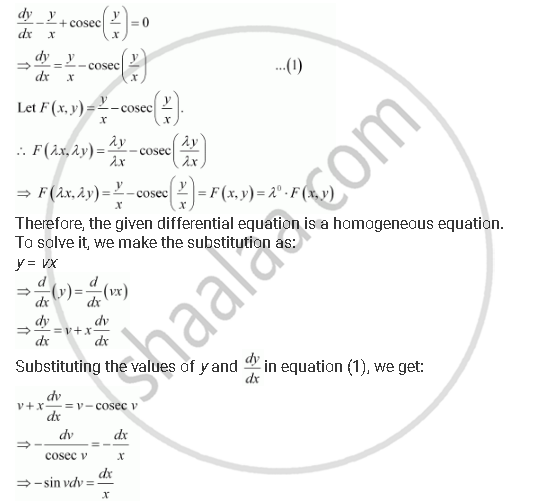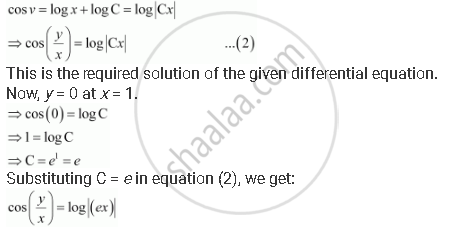Share

# For the Differential Equations Find the Particular Solution Satisfying the Given Condition: Dy/Dx - Y/X + Cosec (Y/X) = 0; Y = 0 When X = 1 - CBSE (Commerce) Class 12 - Mathematics

ConceptMethods of Solving First Order, First Degree Differential Equations Homogeneous Differential Equations

#### Question

For the differential equations find the particular solution satisfying the given condition:

dy/dx -  y/x + cosec (y/x) = 0; y = 0 when x = 1

#### SolutionIntegrating both sides, we get:This is the required solution of the given differential equation.

This is the required solution of the given differential equation.

Is there an error in this question or solution?

#### APPEARS IN

NCERT Solution for Mathematics Textbook for Class 12 (2018 to Current)
Chapter 9: Differential Equations
Q: 14 | Page no. 406
Solution For the Differential Equations Find the Particular Solution Satisfying the Given Condition: Dy/Dx - Y/X + Cosec (Y/X) = 0; Y = 0 When X = 1 Concept: Methods of Solving First Order, First Degree Differential Equations - Homogeneous Differential Equations.
S